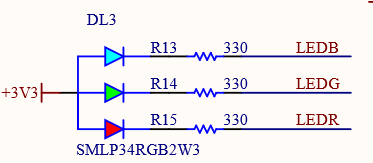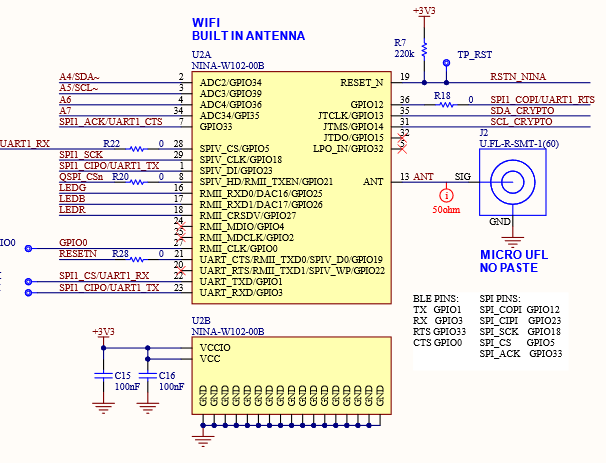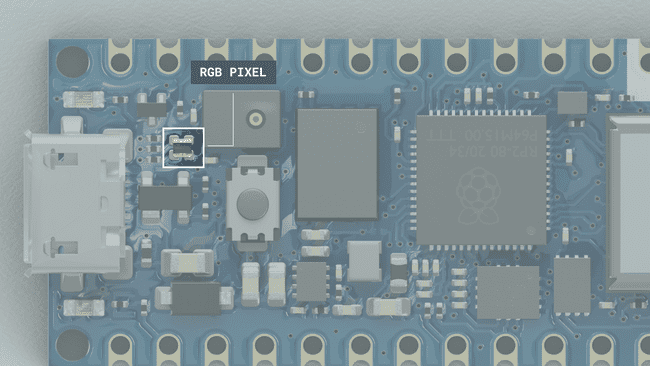Home CodeArduino RGB LED examples on the Arduino Nano RP2040 Connect

# RGB LED examples on the Arduino Nano RP2040 Connect

In this article we look at the RGB led which is fitted on the Arduino Nano RP2040 Connect

The RGB LED (DL3) is a common anode LED that is connected to the Nina W102 module. The LED is off when the digital state is HIGH and on when the digital state is LOW.While using the BLE mode on the NINA module, the RGB cannot be used by default. While the module is in BLE mode, SPI is deactivated, which is used to control the RGBs.

We can see that the RGB led pins are connected to pins 16 to 18 on the UBlox NINA Module. So in order to use the RGB LED, we are going to have to go through this WiFi module.Here is the physical location of this RGB ledParts Required

### Code Examples

You need to install the WifiNINA library

This must be installed from the Library Manager:

• Click Tools -> Manage Libraries to open the Library Manager.
• Search for WifiNINA and install the latest version of the library.

[codesyntax lang=”cpp”]

```#include <WiFiNINA.h>

void setup()
{
// Set LED Pins as Outputs
pinMode(LEDR, OUTPUT);
pinMode(LEDG, OUTPUT);
pinMode(LEDB, OUTPUT);
}

void loop()
{
digitalWrite(LEDR,HIGH); // Turn On RED LED
delay(500);
digitalWrite(LEDR,LOW); // Turn Off RED LED

digitalWrite(LEDG,HIGH); // Turn On GREEN LED
delay(500);
digitalWrite(LEDG,LOW); // Turn Off GREEN LED

digitalWrite(LEDB,HIGH); // Turn On BLUE LED
delay(500);
digitalWrite(LEDB,LOW); // Turn Off BLUE LED
}```

[/codesyntax]

[codesyntax lang=”cpp”]

```#include <WiFiNINA.h>

int rgbRed = 0, dir=1;

void setup()
{
// Set the LED pins as outputs
pinMode(LEDR, OUTPUT);
}

void loop()
{
rgbRed = rgbRed + (1 * dir);

if(rgbRed > 255)
{
rgbRed = 255;
dir = -1;
}

if(rgbRed < 0)
{
rgbRed = 0;
dir = 1;
}
analogWrite(LEDR, rgbRed);
delay(5);
}```

[/codesyntax]

#### Random LED colors

[codesyntax lang=”cpp”]

```#include <WiFiNINA.h>

void setup()
{
// Set the RGB pins to output
pinMode(LEDR, OUTPUT);
pinMode(LEDG, OUTPUT);
pinMode(LEDB, OUTPUT);
randomSeed(1);
}

void loop()
{
// sets the value (range from 0 to 255) for 3 RGB channels:
analogWrite(LEDR, random(255));
analogWrite(LEDB, random(255));
analogWrite(LEDG, random(255));
// wait for 500 milliseconds before changing the color again
delay(500);
}```

[/codesyntax]